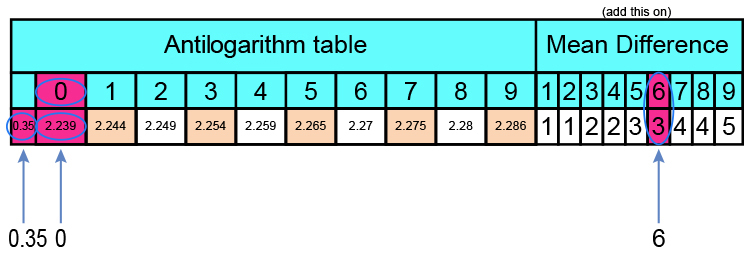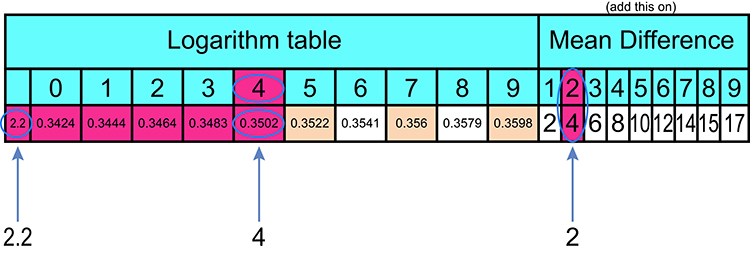# Log tables and antilog tables

Antilog tables have the same numbers in them as log tables - But in reverse

NOTE:

You don’t need antilog tables but if you have them it’s easier to look up.

To prove this

Find the antilog of 10^0.3506

## Using antilogarithm tables2.239 +3 = las\t \ digit = 2.242

10^0.3506 = 2.242

## Now using the reverse of logarithm tables0.3506\ – 0.3502 = las\t \ digit = 4

= 2.242

Conclusion

Same number found but slightly more difficult